# DICS for power mapping#

In this tutorial, we’ll simulate two signals originating from two locations on the cortex. These signals will be sinusoids, so we’ll be looking at oscillatory activity (as opposed to evoked activity).

We’ll use dynamic imaging of coherent sources (DICS)  to map out spectral power along the cortex. Let’s see if we can find our two simulated sources.

```# Author: Marijn van Vliet <w.m.vanvliet@gmail.com>
#
```

## Setup#

We first import the required packages to run this tutorial and define a list of filenames for various things we’ll be using.

```import numpy as np
from scipy.signal import welch, coherence, unit_impulse
from matplotlib import pyplot as plt

import mne
from mne.datasets import sample
from mne.minimum_norm import make_inverse_operator, apply_inverse
from mne.time_frequency import csd_morlet
from mne.beamformer import make_dics, apply_dics_csd

# We use the MEG and MRI setup from the MNE-sample dataset
subjects_dir = data_path / "subjects"

# Filenames for various files we'll be using
meg_path = data_path / "MEG" / "sample"
raw_fname = meg_path / "sample_audvis_raw.fif"
fwd_fname = meg_path / "sample_audvis-meg-eeg-oct-6-fwd.fif"
cov_fname = meg_path / "sample_audvis-cov.fif"

# Seed for the random number generator
rand = np.random.RandomState(42)
```
```Reading forward solution from /home/circleci/mne_data/MNE-sample-data/MEG/sample/sample_audvis-meg-eeg-oct-6-fwd.fif...
Computing patch statistics...
[done]
Computing patch statistics...
[done]
Desired named matrix (kind = 3523) not available
Read MEG forward solution (7498 sources, 306 channels, free orientations)
Desired named matrix (kind = 3523) not available
Read EEG forward solution (7498 sources, 60 channels, free orientations)
Forward solutions combined: MEG, EEG
Source spaces transformed to the forward solution coordinate frame
```

## Data simulation#

The following function generates a timeseries that contains an oscillator, whose frequency fluctuates a little over time, but stays close to 10 Hz. We’ll use this function to generate our two signals.

```sfreq = 50.0  # Sampling frequency of the generated signal
n_samp = int(round(10.0 * sfreq))
times = np.arange(n_samp) / sfreq  # 10 seconds of signal
n_times = len(times)

def coh_signal_gen():
"""Generate an oscillating signal.

Returns
-------
signal : ndarray
The generated signal.
"""
t_rand = 0.001  # Variation in the instantaneous frequency of the signal
std = 0.1  # Std-dev of the random fluctuations added to the signal
base_freq = 10.0  # Base frequency of the oscillators in Hertz
n_times = len(times)

# Generate an oscillator with varying frequency and phase lag.
signal = np.sin(
2.0
* np.pi
* (
base_freq * np.arange(n_times) / sfreq
+ np.cumsum(t_rand * rand.randn(n_times))
)
)

# Add some random fluctuations to the signal.
signal += std * rand.randn(n_times)

# Scale the signal to be in the right order of magnitude (~100 nAm)
# for MEG data.
signal *= 100e-9

return signal
```

Let’s simulate two timeseries and plot some basic information about them.

```signal1 = coh_signal_gen()
signal2 = coh_signal_gen()

fig, axes = plt.subplots(2, 2, figsize=(8, 4))

# Plot the timeseries
ax = axes
ax.plot(times, 1e9 * signal1, lw=0.5)
ax.set(
xlabel="Time (s)", xlim=times[[0, -1]], ylabel="Amplitude (Am)", title="Signal 1"
)
ax = axes
ax.plot(times, 1e9 * signal2, lw=0.5)
ax.set(xlabel="Time (s)", xlim=times[[0, -1]], title="Signal 2")

# Power spectrum of the first timeseries
f, p = welch(signal1, fs=sfreq, nperseg=128, nfft=256)
ax = axes
# Only plot the first 100 frequencies
ax.plot(f[:100], 20 * np.log10(p[:100]), lw=1.0)
ax.set(
xlabel="Frequency (Hz)",
xlim=f[[0, 99]],
ylabel="Power (dB)",
title="Power spectrum of signal 1",
)

# Compute the coherence between the two timeseries
f, coh = coherence(signal1, signal2, fs=sfreq, nperseg=100, noverlap=64)
ax = axes
ax.plot(f[:50], coh[:50], lw=1.0)
ax.set(
xlabel="Frequency (Hz)",
xlim=f[[0, 49]],
ylabel="Coherence",
title="Coherence between the timeseries",
)
fig.tight_layout()
```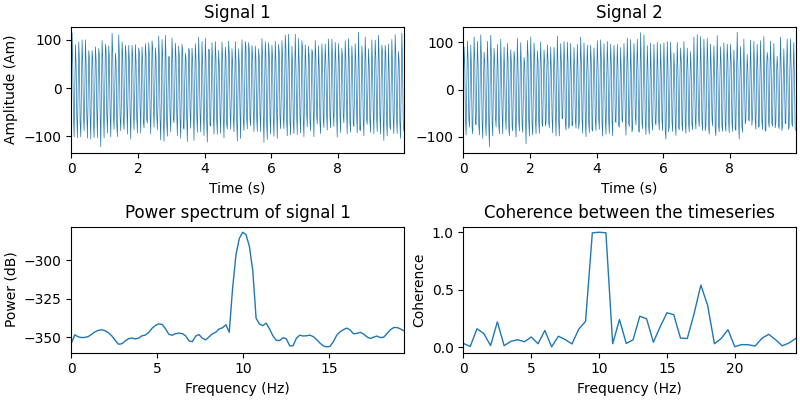Now we put the signals at two locations on the cortex. We construct a `mne.SourceEstimate` object to store them in.

The timeseries will have a part where the signal is active and a part where it is not. The techniques we’ll be using in this tutorial depend on being able to contrast data that contains the signal of interest versus data that does not (i.e. it contains only noise).

```# The locations on the cortex where the signal will originate from. These
# locations are indicated as vertex numbers.
vertices = [, ]

# Construct SourceEstimates that describe the signals at the cortical level.
data = np.vstack((signal1, signal2))
stc_signal = mne.SourceEstimate(
data, vertices, tmin=0, tstep=1.0 / sfreq, subject="sample"
)
stc_noise = stc_signal * 0.0
```

Before we simulate the sensor-level data, let’s define a signal-to-noise ratio. You are encouraged to play with this parameter and see the effect of noise on our results.

```snr = 1.0  # Signal-to-noise ratio. Decrease to add more noise.
```

Now we run the signal through the forward model to obtain simulated sensor data. To save computation time, we’ll only simulate gradiometer data. You can try simulating other types of sensors as well.

Some noise is added based on the baseline noise covariance matrix from the sample dataset, scaled to implement the desired SNR.

```# Read the info from the sample dataset. This defines the location of the
# sensors and such.

picks = mne.pick_types(info, meg="grad", stim=True, exclude=())
mne.pick_info(info, picks, copy=False)

# Define a covariance matrix for the simulated noise. In this tutorial, we use
# a simple diagonal matrix.
cov["data"] *= (20.0 / snr) ** 2  # Scale the noise to achieve the desired SNR

# Simulate the raw data, with a lowpass filter on the noise
stcs = [
(stc_signal, unit_impulse(n_samp, dtype=int) * 1),
(stc_noise, unit_impulse(n_samp, dtype=int) * 2),
]  # stacked in time
duration = (len(stc_signal.times) * 2) / sfreq
raw = simulate_raw(info, stcs, forward=fwd)
add_noise(raw, cov, iir_filter=[4, -4, 0.8], random_state=rand)
```
```Opening raw data file /home/circleci/mne_data/MNE-sample-data/MEG/sample/sample_audvis_raw.fif...
Read a total of 3 projection items:
PCA-v1 (1 x 102)  idle
PCA-v2 (1 x 102)  idle
PCA-v3 (1 x 102)  idle
Range : 25800 ... 192599 =     42.956 ...   320.670 secs
Setting up raw simulation: 1 position, "cos2" interpolation
Event information stored on channel:              STI 014
Interval 0.000–10.000 s
Setting up forward solutions
Computing gain matrix for transform #1/1
Interval 0.000–10.000 s
2 STC iterations provided
[done]
Adding noise to 204/213 channels (204 channels in cov)
```
Measurement date December 03, 2002 19:01:10 GMT MEG Unknown 146 points 204 Gradiometers, 9 Stimulus MEG 2443 Not available Not available 50.00 Hz 0.10 Hz 25.00 Hz PCA-v1 : offPCA-v2 : offPCA-v3 : off 00:00:20 (HH:MM:SS)

We create an `mne.Epochs` object containing two trials: one with both noise and signal and one with just noise

```events = mne.find_events(raw, initial_event=True)
tmax = (len(stc_signal.times) - 1) / sfreq
epochs = mne.Epochs(
raw,
events,
event_id=dict(signal=1, noise=2),
tmin=0,
tmax=tmax,
baseline=None,
)
assert len(epochs) == 2  # ensure that we got the two expected events

# Plot some of the channels of the simulated data that are situated above one
# of our simulated sources.
picks = mne.pick_channels(
epochs.ch_names,
ordered=False,
)
epochs.plot(picks=picks)
```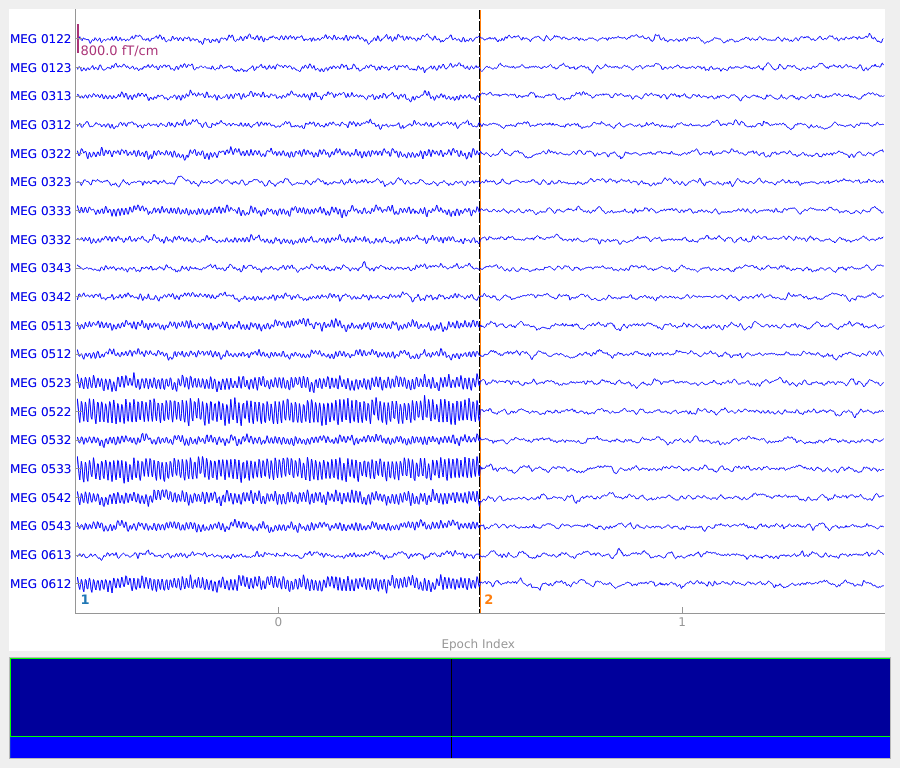```2 events found
Event IDs: [1 2]
2 matching events found
No baseline correction applied
3 projection items activated
Using data from preloaded Raw for 2 events and 500 original time points ...
```

## Power mapping#

With our simulated dataset ready, we can now pretend to be researchers that have just recorded this from a real subject and are going to study what parts of the brain communicate with each other.

First, we’ll create a source estimate of the MEG data. We’ll use both a straightforward MNE-dSPM inverse solution for this, and the DICS beamformer which is specifically designed to work with oscillatory data.

Computing the inverse using MNE-dSPM:

```# Compute the inverse operator
inv = make_inverse_operator(epochs.info, fwd, cov)

# Apply the inverse model to the trial that also contains the signal.
s = apply_inverse(epochs["signal"].average(), inv)

# Take the root-mean square along the time dimension and plot the result.
s_rms = np.sqrt((s**2).mean())
title = "MNE-dSPM inverse (RMS)"
brain = s_rms.plot(
"sample",
subjects_dir=subjects_dir,
hemi="both",
figure=1,
size=600,
time_label=title,
title=title,
)

# Indicate the true locations of the source activity on the plot.

# Rotate the view and add a title.
brain.show_view(azimuth=0, elevation=0, distance=550, focalpoint=(0, 0, 0))
```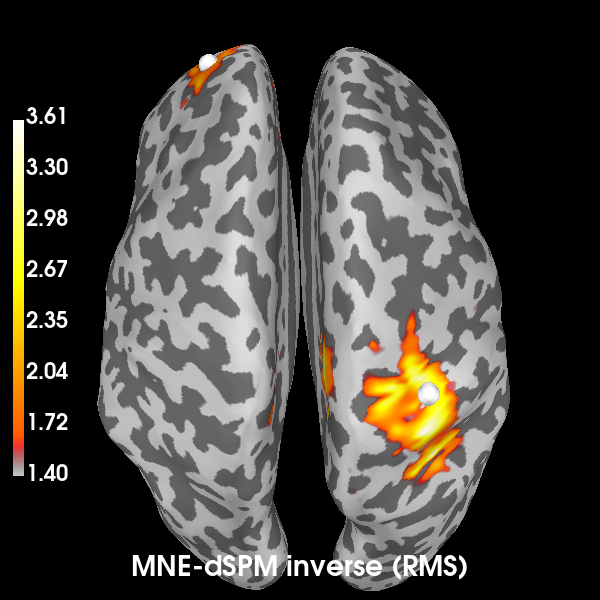```Reading forward solution from /home/circleci/mne_data/MNE-sample-data/MEG/sample/sample_audvis-meg-eeg-oct-6-fwd.fif...
Computing patch statistics...
[done]
Computing patch statistics...
[done]
Desired named matrix (kind = 3523) not available
Read MEG forward solution (7498 sources, 306 channels, free orientations)
Desired named matrix (kind = 3523) not available
Read EEG forward solution (7498 sources, 60 channels, free orientations)
Forward solutions combined: MEG, EEG
Source spaces transformed to the forward solution coordinate frame
Converting forward solution to surface orientation
Average patch normals will be employed in the rotation to the local surface coordinates....
Converting to surface-based source orientations...
[done]
Computing inverse operator with 203 channels.
203 out of 366 channels remain after picking
Selected 203 channels
Creating the depth weighting matrix...
203 planar channels
limit = 7262/7498 = 10.020865
scale = 2.58122e-08 exp = 0.8
Applying loose dipole orientations to surface source spaces: 0.2
Whitening the forward solution.
Computing rank from covariance with rank=None
Using tolerance 4.5e-14 (2.2e-16 eps * 203 dim * 1  max singular value)
GRAD: rank 203 computed from 203 data channels with 0 projectors
Setting small GRAD eigenvalues to zero (without PCA)
Creating the source covariance matrix
Computing SVD of whitened and weighted lead field matrix.
largest singular value = 5.59828
scaling factor to adjust the trace = 2.89697e+18 (nchan = 203 nzero = 0)
NOTE: pick_channels() is a legacy function. New code should use inst.pick(...).
Removing projector <Projection | PCA-v1, active : True, n_channels : 102>
Removing projector <Projection | PCA-v2, active : True, n_channels : 102>
Removing projector <Projection | PCA-v3, active : True, n_channels : 102>
Preparing the inverse operator for use...
Scaled noise and source covariance from nave = 1 to nave = 1
Created the regularized inverter
The projection vectors do not apply to these channels.
Created the whitener using a noise covariance matrix with rank 203 (0 small eigenvalues omitted)
Computing noise-normalization factors (dSPM)...
[done]
Applying inverse operator to "signal"...
Picked 203 channels from the data
Computing inverse...
Eigenleads need to be weighted ...
Computing residual...
Explained  74.8% variance
Combining the current components...
dSPM...
[done]
Using control points [1.40489756 1.65479053 3.61269642]
```

We will now compute the cortical power map at 10 Hz. using a DICS beamformer. A beamformer will construct for each vertex a spatial filter that aims to pass activity originating from the vertex, while dampening activity from other sources as much as possible.

The `mne.beamformer.make_dics()` function has many switches that offer precise control over the way the filter weights are computed. Currently, there is no clear consensus regarding the best approach. This is why we will demonstrate two approaches here:

1. The approach as described in , which first normalizes the forward solution and computes a vector beamformer.

2. The scalar beamforming approach based on , which uses weight normalization instead of normalizing the forward solution.

```# Estimate the cross-spectral density (CSD) matrix on the trial containing the
# signal.
csd_signal = csd_morlet(epochs["signal"], frequencies=)

# Compute the spatial filters for each vertex, using two approaches.
filters_approach1 = make_dics(
info,
fwd,
csd_signal,
reg=0.05,
pick_ori="max-power",
depth=1.0,
inversion="single",
weight_norm=None,
real_filter=True,
)
print(filters_approach1)

filters_approach2 = make_dics(
info,
fwd,
csd_signal,
reg=0.05,
pick_ori="max-power",
depth=None,
inversion="matrix",
weight_norm="unit-noise-gain",
real_filter=True,
)
print(filters_approach2)

# You can save these to disk with:
# filters_approach1.save('filters_1-dics.h5')

# Compute the DICS power map by applying the spatial filters to the CSD matrix.
power_approach1, f = apply_dics_csd(csd_signal, filters_approach1)
power_approach2, f = apply_dics_csd(csd_signal, filters_approach2)
```
```Removing projector <Projection | PCA-v1, active : True, n_channels : 102>
Removing projector <Projection | PCA-v2, active : True, n_channels : 102>
Removing projector <Projection | PCA-v3, active : True, n_channels : 102>
Computing cross-spectral density from epochs...

0%|          | CSD epoch blocks : 0/1 [00:00<?,       ?it/s][Parallel(n_jobs=1)]: Using backend SequentialBackend with 1 concurrent workers.
[Parallel(n_jobs=1)]: Done   1 out of   1 | elapsed:    0.1s remaining:    0.0s
[Parallel(n_jobs=1)]: Done   1 out of   1 | elapsed:    0.1s finished

100%|##########| CSD epoch blocks : 1/1 [00:00<00:00,   13.18it/s]
100%|##########| CSD epoch blocks : 1/1 [00:00<00:00,   13.12it/s]
[done]
Identifying common channels ...
Dropped the following channels:
['EEG 006', 'MEG 1311', 'MEG 1711', 'MEG 1421', 'MEG 1121', 'MEG 0741', 'MEG 1241', 'EEG 054', 'MEG 0321', 'MEG 2231', 'STI 002', 'EEG 033', 'MEG 2221', 'MEG 1411', 'EEG 042', 'MEG 1911', 'EEG 024', 'MEG 0721', 'EEG 017', 'MEG 2131', 'EEG 031', 'MEG 1341', 'MEG 1211', 'MEG 1021', 'EEG 043', 'EEG 060', 'EEG 026', 'EEG 018', 'MEG 2441', 'STI 006', 'EEG 003', 'EEG 041', 'MEG 2443', 'MEG 1321', 'MEG 1531', 'MEG 1621', 'EEG 007', 'MEG 0221', 'EEG 019', 'MEG 1541', 'MEG 2011', 'EEG 009', 'MEG 2241', 'EEG 051', 'MEG 0821', 'EEG 057', 'EEG 040', 'EEG 055', 'MEG 0141', 'MEG 0611', 'EEG 049', 'MEG 0511', 'EEG 002', 'MEG 1131', 'EEG 021', 'MEG 1331', 'MEG 2141', 'MEG 1011', 'MEG 2321', 'EEG 038', 'EEG 010', 'MEG 1831', 'MEG 2431', 'EEG 048', 'EEG 005', 'MEG 2041', 'MEG 2641', 'MEG 0331', 'EEG 014', 'MEG 1231', 'MEG 1941', 'EEG 052', 'EEG 013', 'EEG 053', 'STI 003', 'MEG 0311', 'MEG 2021', 'MEG 2611', 'MEG 2411', 'MEG 1731', 'EEG 023', 'EEG 035', 'MEG 2531', 'MEG 1821', 'MEG 0531', 'MEG 0911', 'MEG 2341', 'EEG 044', 'MEG 2541', 'EEG 046', 'MEG 2031', 'MEG 0121', 'EEG 037', 'MEG 2421', 'EEG 050', 'MEG 0341', 'EEG 015', 'EEG 047', 'STI 016', 'EEG 027', 'MEG 0411', 'MEG 2211', 'MEG 1741', 'STI 004', 'MEG 1221', 'MEG 0441', 'MEG 2621', 'MEG 1521', 'EEG 011', 'MEG 1511', 'EEG 025', 'STI 005', 'MEG 0641', 'EEG 001', 'STI 015', 'EEG 020', 'MEG 1841', 'MEG 0731', 'MEG 0211', 'MEG 2111', 'MEG 0941', 'MEG 0711', 'MEG 1721', 'MEG 0811', 'EEG 008', 'MEG 0521', 'MEG 2311', 'MEG 2331', 'MEG 1931', 'MEG 0541', 'MEG 1921', 'STI 014', 'MEG 0921', 'EEG 034', 'MEG 0431', 'EEG 032', 'MEG 2521', 'EEG 029', 'MEG 1811', 'MEG 0231', 'EEG 036', 'EEG 022', 'MEG 1031', 'MEG 1611', 'MEG 1431', 'EEG 056', 'MEG 0931', 'EEG 039', 'EEG 028', 'EEG 016', 'MEG 0421', 'MEG 1041', 'MEG 0111', 'EEG 004', 'MEG 0131', 'EEG 059', 'EEG 045', 'MEG 1641', 'MEG 1441', 'MEG 0621', 'EEG 012', 'MEG 1141', 'MEG 1111', 'EEG 058', 'MEG 0241', 'STI 001', 'MEG 2121', 'MEG 2511', 'MEG 2631', 'EEG 030', 'MEG 0631', 'MEG 1631']
Computing inverse operator with 203 channels.
203 out of 203 channels remain after picking
Selected 203 channels
Creating the depth weighting matrix...
Whitening the forward solution.
Computing rank from covariance with rank=None
Using tolerance 4.5e+08 (2.2e-16 eps * 203 dim * 1e+22  max singular value)
GRAD: rank 203 computed from 203 data channels with 0 projectors
Setting small GRAD eigenvalues to zero (without PCA)
Creating the source covariance matrix
Computing rank from covariance with rank=None
Using tolerance 1.4e-12 (2.2e-16 eps * 203 dim * 32  max singular value)
GRAD: rank 139 computed from 203 data channels with 0 projectors
Computing DICS spatial filters...
Computing beamformer filters for 7498 sources
Filter computation complete
<Beamformer | DICS, subject "sample", 7498 vert, 203 ch, max-power ori, single inversion>
Identifying common channels ...
Dropped the following channels:
['EEG 006', 'MEG 1311', 'MEG 1711', 'MEG 1421', 'MEG 1121', 'MEG 0741', 'MEG 1241', 'EEG 054', 'MEG 0321', 'MEG 2231', 'STI 002', 'EEG 033', 'MEG 2221', 'MEG 1411', 'EEG 042', 'MEG 1911', 'EEG 024', 'MEG 0721', 'EEG 017', 'MEG 2131', 'EEG 031', 'MEG 1341', 'MEG 1211', 'MEG 1021', 'EEG 043', 'EEG 060', 'EEG 026', 'EEG 018', 'MEG 2441', 'STI 006', 'EEG 003', 'EEG 041', 'MEG 2443', 'MEG 1321', 'MEG 1531', 'MEG 1621', 'EEG 007', 'MEG 0221', 'EEG 019', 'MEG 1541', 'MEG 2011', 'EEG 009', 'MEG 2241', 'EEG 051', 'MEG 0821', 'EEG 057', 'EEG 040', 'EEG 055', 'MEG 0141', 'MEG 0611', 'EEG 049', 'MEG 0511', 'EEG 002', 'MEG 1131', 'EEG 021', 'MEG 1331', 'MEG 2141', 'MEG 1011', 'MEG 2321', 'EEG 038', 'EEG 010', 'MEG 1831', 'MEG 2431', 'EEG 048', 'EEG 005', 'MEG 2041', 'MEG 2641', 'MEG 0331', 'EEG 014', 'MEG 1231', 'MEG 1941', 'EEG 052', 'EEG 013', 'EEG 053', 'STI 003', 'MEG 0311', 'MEG 2021', 'MEG 2611', 'MEG 2411', 'MEG 1731', 'EEG 023', 'EEG 035', 'MEG 2531', 'MEG 1821', 'MEG 0531', 'MEG 0911', 'MEG 2341', 'EEG 044', 'MEG 2541', 'EEG 046', 'MEG 2031', 'MEG 0121', 'EEG 037', 'MEG 2421', 'EEG 050', 'MEG 0341', 'EEG 015', 'EEG 047', 'STI 016', 'EEG 027', 'MEG 0411', 'MEG 2211', 'MEG 1741', 'STI 004', 'MEG 1221', 'MEG 0441', 'MEG 2621', 'MEG 1521', 'EEG 011', 'MEG 1511', 'EEG 025', 'STI 005', 'MEG 0641', 'EEG 001', 'STI 015', 'EEG 020', 'MEG 1841', 'MEG 0731', 'MEG 0211', 'MEG 2111', 'MEG 0941', 'MEG 0711', 'MEG 1721', 'MEG 0811', 'EEG 008', 'MEG 0521', 'MEG 2311', 'MEG 2331', 'MEG 1931', 'MEG 0541', 'MEG 1921', 'STI 014', 'MEG 0921', 'EEG 034', 'MEG 0431', 'EEG 032', 'MEG 2521', 'EEG 029', 'MEG 1811', 'MEG 0231', 'EEG 036', 'EEG 022', 'MEG 1031', 'MEG 1611', 'MEG 1431', 'EEG 056', 'MEG 0931', 'EEG 039', 'EEG 028', 'EEG 016', 'MEG 0421', 'MEG 1041', 'MEG 0111', 'EEG 004', 'MEG 0131', 'EEG 059', 'EEG 045', 'MEG 1641', 'MEG 1441', 'MEG 0621', 'EEG 012', 'MEG 1141', 'MEG 1111', 'EEG 058', 'MEG 0241', 'STI 001', 'MEG 2121', 'MEG 2511', 'MEG 2631', 'EEG 030', 'MEG 0631', 'MEG 1631']
Computing inverse operator with 203 channels.
203 out of 203 channels remain after picking
Selected 203 channels
Whitening the forward solution.
Computing rank from covariance with rank=None
Using tolerance 4.5e+08 (2.2e-16 eps * 203 dim * 1e+22  max singular value)
GRAD: rank 203 computed from 203 data channels with 0 projectors
Setting small GRAD eigenvalues to zero (without PCA)
Creating the source covariance matrix
Computing rank from covariance with rank=None
Using tolerance 1.4e-12 (2.2e-16 eps * 203 dim * 32  max singular value)
GRAD: rank 139 computed from 203 data channels with 0 projectors
Computing DICS spatial filters...
Computing beamformer filters for 7498 sources
Filter computation complete
<Beamformer | DICS, subject "sample", 7498 vert, 203 ch, max-power ori, unit-noise-gain norm, matrix inversion>
Computing DICS source power...
[done]
Computing DICS source power...
[done]
```

Plot the DICS power maps for both approaches, starting with the first:

```def plot_approach(power, n):
"""Plot the results on a brain."""
title = "DICS power map, approach %d" % n
brain = power_approach1.plot(
"sample",
subjects_dir=subjects_dir,
hemi="both",
size=600,
time_label=title,
title=title,
)
# Indicate the true locations of the source activity on the plot.
# Rotate the view and add a title.
brain.show_view(azimuth=0, elevation=0, distance=550, focalpoint=(0, 0, 0))
return brain

brain1 = plot_approach(power_approach1, 1)
```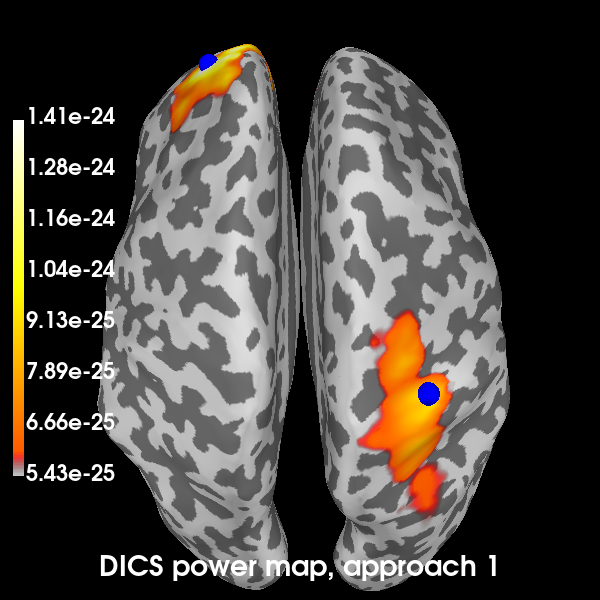```Using control points [5.42918831e-25 6.04345299e-25 1.40589757e-24]
```

Now the second:

```brain2 = plot_approach(power_approach2, 2)
```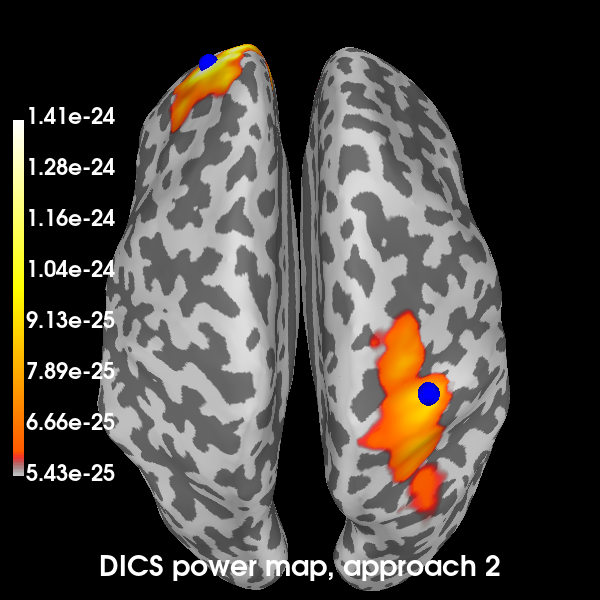```Using control points [5.42918831e-25 6.04345299e-25 1.40589757e-24]
```

Excellent! All methods found our two simulated sources. Of course, with a signal-to-noise ratio (SNR) of 1, is isn’t very hard to find them. You can try playing with the SNR and see how the MNE-dSPM and DICS approaches hold up in the presence of increasing noise. In the presence of more noise, you may need to increase the regularization parameter of the DICS beamformer.